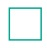Home | | Maths 6th Std | Exercise 3.3

# Exercise 3.3

6th Maths : Term 1 Unit 3 : Ratio and Proportion : Exercise 3.3 : Text Book Back Exercises Questions with Answers, Solution

Exercise 3.3

1. Fill in the boxes.

(i) 3 : 5 : : ___ : 20 Answer: 12

(ii): 24 :: 3 : 8 Answer: 9

(iii) 5 :10 : 8 :: 15:Answer: 4, 12

(iv) 12 :=: 4 = 8 : 16 Answer: 24, 2

2. Say True or False.

(i) 2 : 7 :: 14 : 4 [False]

(ii) 7 Persons is to 49 Persons as 11 kg is to 88 kg [False]

(iii) 10 books is to 15 books as 3 books is to 15 books [False]

3. Using the numbers 3, 9, 4, 12 write two ratios that are in proportion.

Solution:

Using numbers = 3, 9, 4, 12

Ratios in proportion

(i) 3 : 9 : : 4 : 12

(ii) 9:3:: 12: 4

4. Find whether 12, 24, 18, 36 in that order can be expressed as two ratios that are in proportion.

Using numbers = 12, 24, 18, 36

Two ratios =  (i) 12 : 24

(ii) 18:36

Proportion :− 12 : 24 :: 18 : 36

5. Write the mean and extreme terms in the following ratios and check whether they are in proportion.

(i) 78 litres is to 130 litre and 12 bottles is to 20 bottles

(ii) 400 gm is to 50 gm and 25 rupees is to 625 rupees

Solution:

(i) 78: 130:: 12:20

extreme = 78 × 20 = 1560

mean = 130 × 12 = 1560

extreme = mean

Ratios are in proportion

(ii) 400 : 50 : : 25 : 625

extreme = 400 × 625 = 250000

mean = 50 × 25 = 1250

extreme mean

Ratios are not in proportion

6. The America’s famous Golden Gate bridge is 6480 ft long with 756 ft tall towers. A model of this bridge exhibited in a fair is 60 ft long with 7 ft tall towers. Is the model in proportion to the original bridge?Solution:

Bridge Ratio = 6480:756

Model Ratio = 60 : 7

proportion = 6480 : 756 :: 60 : 7

extreme = 6480 × 7 = 45360

mean = 756 × 60 = 45360

extreme = mean

The model is in proportion.

Objective Type Questions

7. Which of the following ratios are in proportion?

(a) 3 : 5 , 6 : 11

(b) 2 : 3, 9 : 6

(c) 2 : 5, 10 : 25

(d) 3 : 1, 1 : 3

Answer: (c) 2 : 5, 10 : 25

8. If the ratios formed using the numbers 2, 5, x, 20 in the same order are in proportion, then x is

(a) 50

(b) 4

(c) 10

(d) 8

9. If 7 : 5 is in proportion to x : 25, then x is

(a) 27

(b) 49

(c) 35

(d) 14

Exercise 3.3

1) (i) 12 (ii) 9 (iii) 4; 12 (iv) 24; 2

2) (i) False (ii) False (iii) False

3) (i) 3 : 9 : :4:12 (ii) 9 : 3 : :12:4

4) Yes

5) (i) Yes (ii) No

6) Yes

7) (c) 2 : 5 , 10 : 25

8) (d) 8

9) (c) 35

Tags : Questions with Answers, Solution | Proportion | Term 1 Chapter 3 | 6th Maths , 6th Maths : Term 1 Unit 3 : Ratio and Proportion
Study Material, Lecturing Notes, Assignment, Reference, Wiki description explanation, brief detail
6th Maths : Term 1 Unit 3 : Ratio and Proportion : Exercise 3.3 | Questions with Answers, Solution | Proportion | Term 1 Chapter 3 | 6th Maths